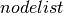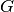Warning

This documents an unmaintained version of NetworkX. Please upgrade to a maintained version and see the current NetworkX documentation.

# to_pandas_dataframe¶

to_pandas_dataframe(G, nodelist=None, multigraph_weight=<built-in function sum>, weight='weight', nonedge=0.0)[source]

Return the graph adjacency matrix as a Pandas DataFrame.

Parameters: G (graph) – The NetworkX graph used to construct the Pandas DataFrame. nodelist (list, optional) – The rows and columns are ordered according to the nodes in. Ifis None, then the ordering is produced by G.nodes(). multigraph_weight ({sum, min, max}, optional) – An operator that determines how weights in multigraphs are handled. The default is to sum the weights of the multiple edges. weight (string or None, optional) – The edge attribute that holds the numerical value used for the edge weight. If an edge does not have that attribute, then the value 1 is used instead. nonedge (float, optional) – The matrix values corresponding to nonedges are typically set to zero. However, this could be undesirable if there are matrix values corresponding to actual edges that also have the value zero. If so, one might prefer nonedges to have some other value, such as nan. df – Graph adjacency matrix Pandas DataFrame

Notes

The DataFrame entries are assigned to the weight edge attribute. When an edge does not have a weight attribute, the value of the entry is set to the number 1. For multiple (parallel) edges, the values of the entries are determined by the ‘multigraph_weight’ parameter. The default is to sum the weight attributes for each of the parallel edges.

Whendoes not contain every node in, the matrix is built from the subgraph ofthat is induced by the nodes in.

The convention used for self-loop edges in graphs is to assign the diagonal matrix entry value to the weight attribute of the edge (or the number 1 if the edge has no weight attribute). If the alternate convention of doubling the edge weight is desired the resulting Pandas DataFrame can be modified as follows:

>>> import pandas as pd
>>> import numpy as np
>>> G = nx.Graph([(1,1)])
>>> df = nx.to_pandas_dataframe(G)
>>> df
1
1  1
>>> df.values[np.diag_indices_from(df)] *= 2
>>> df
1
1  2


Examples

>>> G = nx.MultiDiGraph()